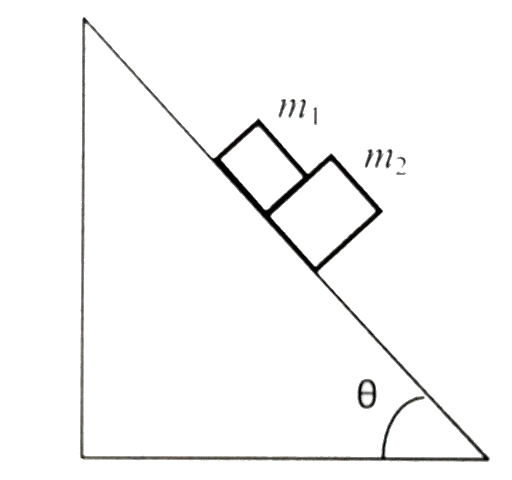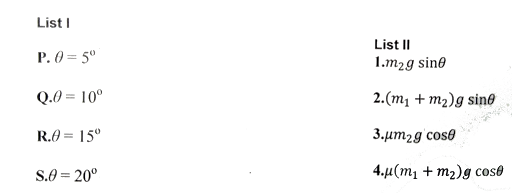A block of mass m1 = 1 kg another mass m2 = 2 kg, are placed toge | Filoclass 11

Missing544

A block of mass m1 = 1 kg another mass m2 = 2 kg, are placed together (see figure) on an inclined plane with angle of inclination . Various values of are given in List I. The coefficient of friction between the block and the plane is always zero. The coefficient of static and dynamic friction between the block and the plane are equal to . In List II expressions for the friction on block are given. Match the correct expression of the friction in List II with the angles given in List I, and choose the correct option. The acceleration due to gravity is denoted by g
[Useful information ].544Connecting you to a tutor in 60 seconds.